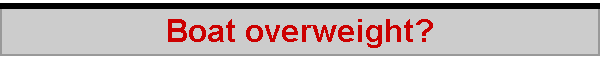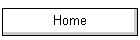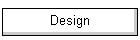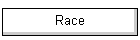I was wondering whether it really mattered if a yacht was a little overweight.  I've taken the "Acceleration" page spreadsheet and produced a whole new version.  It calculates drag and acceleration against a time line, rather than against a speed line (spreadsheet here, approx 96 kb), in order to yield relatively stable calculations.  More importantly, it now factors in the yacht displacement in order to calculate acceleration, and calculates the distance travelled during acceleration.  For a given wind speed and displacement, the sorts of results it gives are shown below, plotting speed and distance against time while reaching and while running.  These particular graphs are for a wind speed of around "1", showing the yacht reaches maximum reaching speed after about "6" units of time.  These are arbitrary units, because the spreadsheet is not calibrated.  However, if you think "1 metre per second" for the wind speed, and "6 seconds" for the time, you'll not be too far out.### Acceleration for various wind speeds

Calculating how long it takes to reach maximum speed is fairly easy, and the first graph below is similar to that given in the previous "Acceleration" and "VPP" Web pages.  What is now more interesting is the second of these graphs, plotting the distance covered while getting up to maximum speed.  A bit of a surprise here -- the yacht travels roughly the same distance no matter the wind speed, to reach maximum speed on the run, about 0.4 units of distance.  While reaching, on the other hand, distance to max speed gets shorter as wind speed increases, from about 3.4 units at low wind, down to about 1.4 units at higher wind speed.  That's more like it.  Again, these are quite arbitrary units, but if you read the distances as "metres", you'd probably be OK.

I have to emphasise, though, that the spreadsheet is just a rough guide.  In particular, it calculates the distance covered against the best advice of Zeno's paradox.  You'll remember the tortoise and the hare and the fact that the hare could never overtake the tortoise because for every inch the hare caught up the tortoise was a half-inch further on.  Turns out that calculating distance covered under acceleration has a similar paradox at its heart if the scenario is cut into little time slices, as the spreadsheet does.### Different displacements

The point of the spreadsheet, though, is to estimate and compare what happens if the yacht's displacement is, say, 4.1 units instead of 4 units.  Yeah, alright, you can think of this as 4 kg and 4.1 kg, but remember, the spreadsheet isn't calibrated...

Here is the result, for the two displacements mentioned, 4.0 vs 4.1.  It is clear that we are talking about very small differences indeed.  At a wind speed of 1, say, where the yacht might take 7 time units to get up to maximum speed reaching, the 4.1 unit displacement yacht ends up around 0.045 units of distance behind -- alright, call it 45 millimetres or just under two inches...!  Now that is a very big surprise.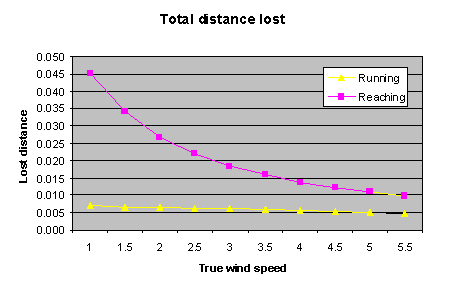What seems to be happening is that the lighter yacht reaches top speed just a little quicker, and only reaches a top speed just a very little higher than the heavier yacht.  So although the heavier yacht might take an extra 0.17 seconds to get there, the lighter yacht wasn't going that much faster in that time, it was stuck at its maximum.  And these calculations are for a standing start.  In reality, the yachts are always travelling near top speed, and so the difference between two different displacements will be even smaller. Negligible, in fact.

Playing with the spreadsheet suggests that, if we imagine a 4 kg IOM pitted against a 4.2 kg IOM (ie seriously overweight by half a pound), and have them tack 10 times up the beat, such that on each tack they lose half their speed and must accelerate again, with a wind speed of 2 m/s, then the heavier yacht will be a mere 20 cm or 8 inches behind.  Wow!  It is certain that other factors will totally overwhelm this small difference.

### Some notes on using the spreadsheet

Some 40 rows are given on the spreadsheet to cover the time period of interest.  The first row starts at time 0, and then each subsequent row has the time increased by the value of the "time increment", 0.2 in the case of the default spreadsheet with a displacement of 4 and a wind speed of 1.

On each row, that is for each time slice, the spreadsheet estimates the drag on the hull, estimates the drive force, estimates the acceleration, and then figures out boat speed and distance travelled while reaching, and while running.  When the accelerating force falls below a small percentage, called "infinitessimal", of the drag force, the spreadsheet decides that maximum speed has been reached, and notes this, along with distance travelled so far.

In fact, the calculations, while quite correct, are both approximate and crude, so the distance results are quite sensitive to the time increment chosen, and to the "infinitessimal" percentage value used.  The speed results are much more reliable.

In order to compare two displacements, the spreadsheet macro changes the skin friction for the higher displacement, on the assumption that the higher displacement incurs an immersed area penalty.  Normally, the "friction multiplier" should be set to 1, and the spreadsheet does this.  When you click on the "Compare displacement" button, having calculated the results for the lighter displacement and copied them over to a second table, the spreadsheet then sets the friction multiplier to the ratio of the lighter to the heavier displacement, and calculates the results for the heavier displacement.  In fact the multiplier is set to the 2/3rds power of the ratio, since in general terms surface area varies as the 2/3rds power of volume.

The spreadsheet works with macros, so don't be surprised if your anti-virus software asks you if you are sure you want to activate 'em.

2005-12-18